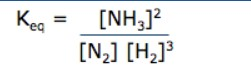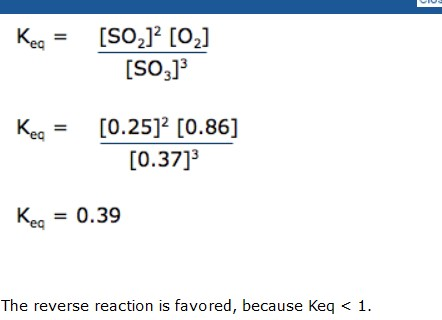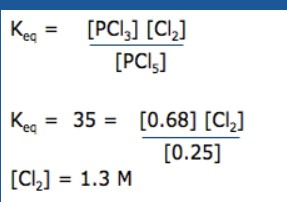# Self Assessment and Practice

When a reaction is at equilibrium, what two things are equal?

The rates of the forward and reverse reactions.

When equilibrium is reached, the concentration of reactants
remains the same
increases
decreases
When equilibrium is reached, the concentration of products
remains the same
increases
decreases

What does the value (the number) of Keq tell you?

If Keq > 1, the forward reaction is favored. If Keq = 1, neither reaction is favored. If Keq < 1, the reverse reaction is favored.

Write a balanced equation for the synthesis of ammonia from its elements, including the word “energy” as a product:

N2 + 3H2 →2NH3 + energy

Write the Keq expression for the reaction above:For the reaction, 2SO3 ↔ 2SO2 + O2, [SO3]=0.37M, [SO2]=0.25M, [O2]=0.86M. Write the Keq equation, calculate Keq, and explain what this value means.For the equilibrium system PCl5 ↔ PCl3 + Cl2, Keq = 35. If the concentrations of PCl5 and PCl3 are 0.025M and 0.68M respectively, what is the concentration of the Cl2?source